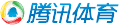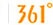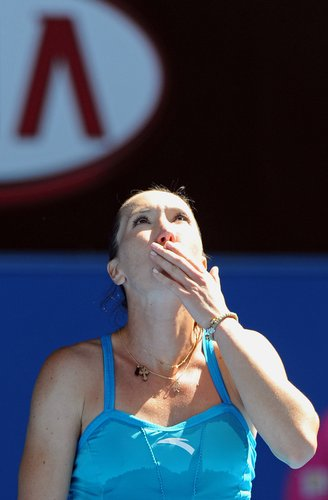腾讯体育 > 新腾讯网球 > WTA新闻 > 正文

# 澳网伊万完胜扬科惊魂 塞尔维亚双姝携手晋级

2010年01月19日13:53腾讯体育我要评论(0)伊万诺维奇vs佩里技术统计 (6-2,6-3) 技术统计 伊万诺维奇(塞尔维亚) 佩里(美国) 一发成功率 32 of 54 = 59 % 30 of 61 = 49 % ACE球 3 0 双误 5 4 非受迫性失误 24 26 一发得分率 26 of 32 = 81 % 19 of 30 = 63 % 二发得分率 10 of 22 = 45 % 10 of 31 = 32 % 制胜分(包括发球直得) 18 17 接发球得分率 32 of 65 = 49 % 18 of 59 = 31 % 破发成功率 5 of 11 = 45 % 2 of 9 = 22 % 网前得分率 15 of 22 = 68 % 15 of 24 = 63 % 总得分 68 47 最快发球时速 172 KMH 181 KMH 一发平均时速 154 KMH 158 KMH 二发平均时速 136 KMH 135 KMH

(小卡)

 扬科维奇vs尼楚勒索技术统计 (6-4,6-0) 技术统计 扬科维奇(塞尔维亚) 尼楚勒索(罗马尼亚) 一发成功率 44 of 62 = 71 % 32 of 47 = 68 % ACE球 3 0 双误 4 0 非受迫性失误 29 33 一发得分率 28 of 44 = 64 % 9 of 32 = 28 % 二发得分率 7 of 18 = 39 % 7 of 15 = 47 % 制胜分(包括发球直得) 16 11 接发球得分率 31 of 47 = 66 % 27 of 66 = 41 % 破发成功率 7 of 7 = 100 % 3 of 8 = 38 % 网前得分率 13 of 26 = 50 % 11 of 16 = 69 % 总得分 66 43 最快发球时速 163 KMH 152 KMH 一发平均时速 152 KMH 141 KMH 二发平均时速 129 KMH 107 KMH

(小卡)

vicar 13:47:34人在热爱
• 打印
• 手机看新闻
• 分享到空间
• 复制链接

• ·
• ·
• ·
• ·
• ·
• ·
• ·
• ·